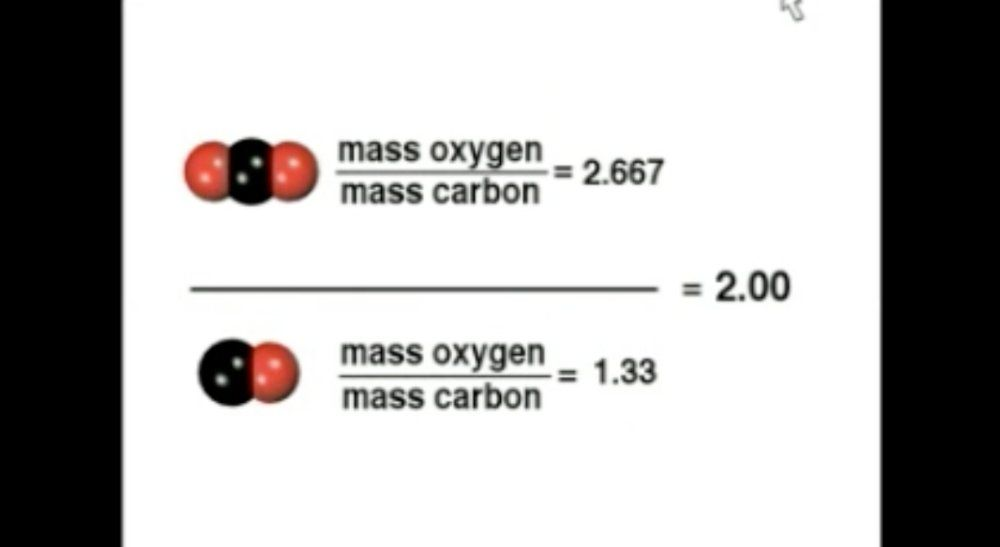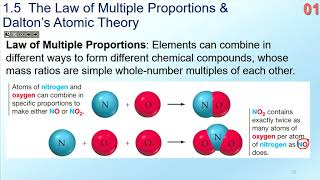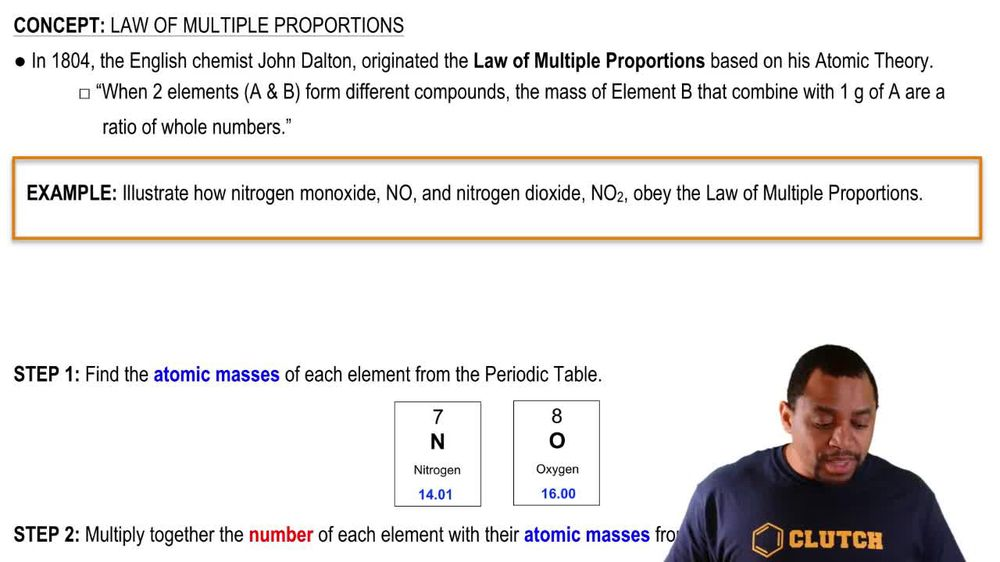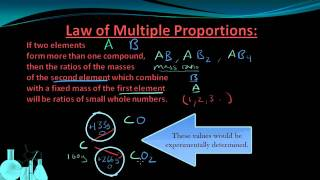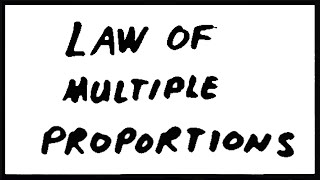Start typing, then use the up and down arrows to select an option from the list.
1. 2. Atoms & Elements2. Law of Multiple Proportions
Problem

# Two compounds containing carbon and oxygen have the following percent composition by mass. Compound 1: 42.9% carbon and 57.1% oxygen Compound 2: 27.3% carbon and 72.7% oxygen Show that the law of multiple proportions is followed. If the formula of the first compound is CO, what is the formula of the second compound?

Relevant Solution2m
Play a video:
give the following problem. Given the following data. Compound one and compound to has the percentages for each adam demonstrate the law of multiple proportions by determining the formula of compound two. When compound one is nitric oxide compound, wanted to only contain nitrogen and oxygen. So the first thing that we want to do is we want to assume that we are working With g for our samples. So we want to assume that working with 100 g for each of our samples. With this in mind, we can find out the oxygen to nitrogen mass ratio for each compound. So for compound one the oxygen to nitrogen ratio is equal to 73 .54g of oxygen over 26.46 g of nitrogen. What we simply did is we took 100 and we multiplied it. Are we divided by 100% to get decimal points. And so we did this year we got 2.779 as our ratio. And for a compound to for our oxygen nitrogen ratio, we got 89.29 grams of oxygen over 10. g of nitrogen to get 8.337. Thirdly we're going to take the oxygen nitrogen Ratio for compound one and divide that ratio by the ratio of compound two. And so the mass ratio for compound two was 8.337. As we solved here. And for compound one, the mass ratio was 2.779. This gives us roughly 3/1 will be simplified. And so therefore we can conclude That when compound one is nitric oxide, N. O. The formula for compound to the formula for compound to is N 03 nitrogen trioxide. And so this is going to be our final answer. Overall, I hope this helped until next time.